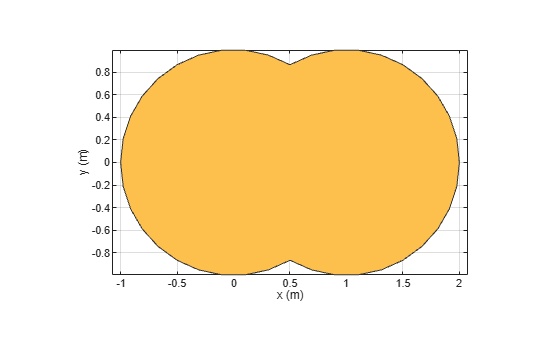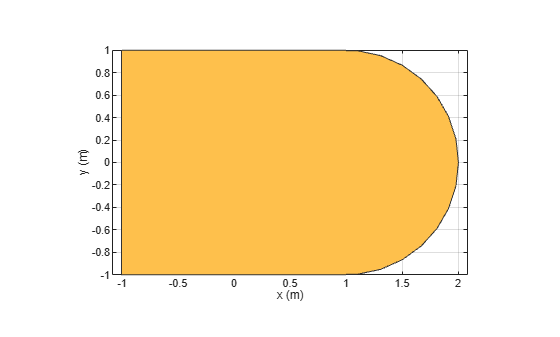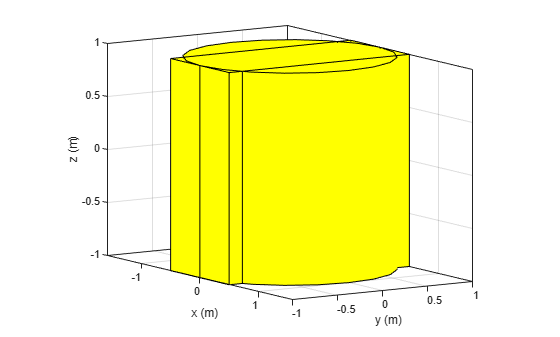Boolean unite operation on two shapes

## Syntax

``add(shape1,shape2)``
``add(shape1,shape2,RetainShape=0)``
``c = add(shape1,shape2)``
``c = add(shape1,shape2,RetainShape=0)``

## Description

example

````add(shape1,shape2)` unites the 2-D or 3-D `shape1` and `shape2` using the add operation retaining the individual shape boundaries and plots the resultant 2-D or 3-D shape. Adjust the `Transparency` property of the shapes to view the retained individual shape portion in the resultant shape. Alternatively, you can also use the `'+'` operator to add the two shapes together.```
````add(shape1,shape2,RetainShape=0)` unites the 3-D `shape1` and `shape2` using the add operation by merging boundaries of both the shapes and plots the resultant 3-D shape.```

example

````c = add(shape1,shape2)` unites the 2-D or 3-D `shape1` and `shape2` using the add operation while retaining the individual shape boundaries and returns a polygon object for the resultant 2-D shape or a custom 3-D object for the resultant 3-D shape. Adjust the `Transparency` property of the shapes to view the retained individual shape portion in the resultant shape. Alternatively, you can also use the `'+'` operator to add the two shapes together.```
````c = add(shape1,shape2,RetainShape=0)` unites the 3-D `shape1` and `shape2` using the add operation by merging boundaries of both the shapes and returns a custom 3-D object for the resultant 3-D shape.```

## Examples

collapse all

Create and view a default circle.

`circle1 = antenna.Circle;`

Create a circle with a radius of 1 m. The center of the circle is at [1 0].

`circle2 = antenna.Circle(Center=[1 0],Radius=1);`

`add(circle1,circle2)`Create a circle with a radius of 1m. The center of the circle is at [1 0].

`circle1 = antenna.Circle(Center=[1 0],Radius=1);`

Create a rectangle with a length of 2m and a width of 4m centered at the origin.

`rect1 = antenna.Rectangle(Length=2,Width=2);`

Add the two shapes together using the `+` function.

`polygon1 = circle1+rect1`
```polygon1 = Polygon with properties: Name: 'mypolygon' Vertices: [21x3 double] ```
`show(polygon1)`This example shows how to add two 3-D shapes and view the combined shape.

Create a box and a cylindrical shape.

```b = shape.Box; c = shape.Cylinder; j = add(b,c)```
```j = Custom3D with properties: Name: 'custom3D' Vertices: [100x3 double] Metal: 'PEC' Dielectric: 'Air' Color: 'Individual' Transparency: 'Individual' EdgeColor: 'Individual' ```

Alternatively, you can use `'+'` operator to add these shapes.

`b + c; `## Input Arguments

collapse all

2-D and 3-D geometric shapes created using shape objects in Custom 2-D and 3-D Antenna.

Example: `antenna.Rectangle,antenna.Circle`

Example: `shape.Box,shape.Custom3D`

## Output Arguments

collapse all

Shape obtained after uniting two 2-D or 3-D shapes.

Example: `antenna.Polygon`

Example: `shape.Polygon`

Example: `shape.Custom3D`

## Version History

Introduced in R2017a